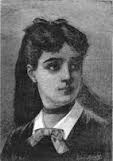# The Conjecture of Marie-Sophie GermainNumbers pervade our lives in many different venues.  Prime numbers, in particular, weave their way into the very fabric of our daily existence.   From surfing the internet to pseudo-random number generators, primes are found ever present behind a multitude of abstractions.    A prime number is a positive integer such that it is only divisible by one and itself.  In light of this definition, the number one is not considered a prime.

Though the properties of prime numbers are well defined, they are still very much mysterious.    For instance, it becomes very ambiguous to calculate where a prime number will occur in a set of very large integers in a sequence.   Around 300 B.C., Euclid proved that there exists infinitely many prime numbers.  This was not intuitive at first, because as numbers become larger, prime numbers become more “rare”.  His proof is simple.  Basically, he assumed that there exists a last prime number$P$.   Then, he further reasoned, that$P$ and all of the prime numbers less than$P$ multiplied together form a composite number$N$.   Therefore, he added one to$N$,$N+1$, to form a new integer that is not divisible by any prime number less than it.  Consequently, this shows that$N+1$ is a prime number by definition, which is proof by contradiction that there exist infinitely many primes.

Many famous open problems in Number Theory (a major subfield of mathematics) are related to the nature of prime numbers.   The nineteenth century French mathematician Marie-Sophie Germain was an originator of one such problem.    She postulated a conjecture that was related to a special set of prime numbers that later became known as Sophie-Germain Primes.  A Sophie-Germain Prime is a prime number$P$ such that$2P+1$ is also a prime.  For example,$2$ is a prime and$2(2)+1 = 5$  is also a prime, making$2$ a Sophie-Germain Prime.    Like Euclid with the regular prime numbers, the conjecture poses the question:  Are there infinitely many Sophie-Germain Primes?

A quick search on the internet will yield the current research and computational results related to the conjecture.    It would be interesting to see the emergence of a clever elementary proof like the one Euclid provided for the infinitude of regular primes.  One could imagine the elegance of such a solution.  How would you approach it?